Teaching support from the UK’s largest provider of in-school maths tuitionone to one lessonsschools supported

Built by teachers for teachers

In-school online one to one maths tuition developed by maths teachers and pedagogy experts

Find out more

Hundreds of FREE online maths resources!

Daily activities, ready-to-go lesson slides, SATs revision packs, video CPD and more!Explore all resources
Maths Mastery

Built by teachers for teachers

In-school online one to one maths tuition developed by maths teachers and pedagogy experts

Find out more

# White Rose Maths Year 5: What Students Learn And The Resources To Support Them

Here we look at the White Rose Maths Year 5 scheme of learning, the topics it covers across the year and provide lots of sample questions and White Rose Maths resources for those following White Rose in Year 5.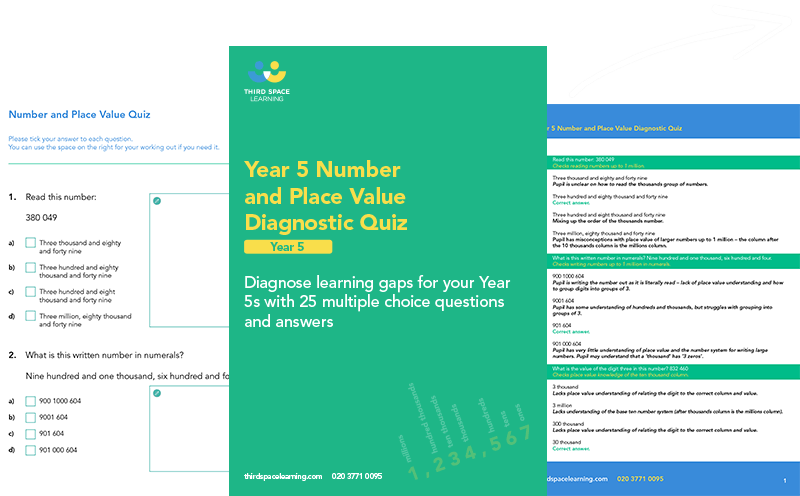Number and Place Value Diagnostic Quiz Year 5

Download this free number and place value diagnostic quiz for year 5 to identify gaps and growth in learning.Download Free Now!

The White Rose Maths scheme of learning follows the national curriculum with a mastery approach to maths with a range of fluency, reasoning and problem solving elements. They also encourage concrete, pictorial and abstract representations to develop deep understanding of mathematical concepts.

It is thought that approximately 70% of schools in the UK follow the White Rose Maths scheme and this is reflected in the thousands of students Third Space Learning tutors every week. Our tutors are highly skilled maths and pedagogy experts, well trained in the language, representations and approach used by White Rose. They are therefore able to deliver one to one maths lessons and interventions that feels familiar to students who are used to White Rose.

Throughout this article you’ll see example lesson slides and example questions from Third Space Learning’s Year 5 maths curriculum that exemplify best practice teaching to the White Rose Maths Year 5 scheme.

We’ve also provided lots of links to White Rose Maths Year 5 resources that are suitable for use for each topic. In addition to the links provided, Third Space Learning also offers libraries for White Rose Maths Year 1, White Rose Maths Year 2, White Rose Maths Year 3, White Rose Maths Year 4 and White Rose Maths Year 6

## About the White Rose Maths Year 5 Scheme of Learning

The primary school White Rose Maths scheme of learning has gone through several iterations and versions up to the current version 3. The scheme of learning SOL is designed as guidance and it is not necessarily intended for each small step to take one lesson. As with all teaching, it is important that teachers adapt this SOL for their class/ pupils.

### Topics covered in Year 5 White Rose Maths scheme of learning work

The White Rose Maths Year 5 SOL outlines which topics are taught, and when, throughout the autumn, spring and summer terms of Year 5.

The breakdown for Version 3 of the scheme of learning is as follows

#### Year 5 autumn term

• Place value
• Multiplication and division A
• Fractions A

#### Year 5 spring term

• Multiplication and division B
• Fractions B
• Decimals and percentages
• Perimeter and area
• Statistics

#### Year 5 summer term

• Shape
• Position and direction
• Decimals
• Negative numbers
• Converting units
• Volume

​​Third Space Learning has created several collections of White Rose Maths aligned resources for all primary year groups from early years through to Year 6. These resources can provide a different perspective on a topic and so help pupils spend the time needed to fully embed a concept.

Here is a summary of the different collections available for schools following Year 5 White Rose Maths, many of them are free to download from our maths hub and some are premium resources. Links to the topic specific versions are provided within each termly breakdown below:

These ready-to-go lesson slides come in the form of editable powerpoints with related worksheets that are designed for everyday teaching. The slides cover both version 2 of the White Rose scheme of learning (in blue) and version 3 of the SOL. They also include support slides that can be used to support pupils who need a little extra  pre/ post teaching to support their understanding.

Code Crackers

Our Code Crackers resources are aligned to the version 2 SOL. These are summative resources designed as a retrieval activity for the end of the place value block.

Maths Code Crackers Year 5

Pre and Post Diagnostic Questions

These tests can be used before starting the place value block to assess gaps, and after teaching the place value block to identify progress.

Diagnostic Assessments Year 5

Worked Examples

Pupils check over completed questions to identify errors (or identify correct answers). Pupils are encouraged to explain the errors they find, not just ‘mark’ work. These worked examples help to solidify understanding by getting the pupil to discuss errors and how to avoid them.

Year 5 Worked Examples

Rapid Reasoning

These reasoning problems go alongside our Fluent in Five resource. One question each week has a model answer so teachers can understand what the question is asking and how to solve it.

Rapid Reasoning Year 5

All Kinds of Word Problems

Test your class’ problem solving skills with these place value word problems.

All Kinds of Word Problems Year 5

## White Rose Maths Year 5 autumn term

In the autumn term, Year 5 focuses on place value, addition & subtraction, multiplication  & division and fractions.

### Place Value Year 5

By the time pupils reach Year 5, place value continues to be the first concept covered, but with a shorter length of time spent on it than in previous years. In autumn block 1 students work with increasingly larger numbers; reading, writing, comparing and ordering numbers to 1,000,000 and continue to develop their understanding of Roman numerals. Pupils build on their understanding of rounding; round to the nearest 10, 100 and 1000.

#### Example year 5 place value questions

1. What is the value of the underlined digit in each number below?

a)534,641 b) 718,076 c) 921,759

1. 6 hundreds or 600
2. 7 tens or 70
3. 20 thousands or 20,000

2. Round 52,532 to the nearest 10, 100 and 1000.

52,532 to the nearest 10 = 52,530

52,532 to the nearest 100 = 52,500

52,532 to the nearest 1,000 = 53,000

### Addition and Subtraction Year 5

In Year 5 autumn block 2, pupils continue to practise using formal written methods of columnar addition and subtraction with increasingly large numbers over 4-digits. Students also develop their checking skills, through rounding and using inverse operations. By this stage pupils are also working on increasingly complex, multi-step word problems.

#### Example year 5 addition and subtraction questions:

1. 52,378 + 87,659 =

2. 86,143 – 48,967 =

### Multiplication and division Year 5

Multiplication and division is split into two blocks. It  is covered initially in the autumn block 3, in Block A and continues with Block B in spring block 1.

### Multiplication and Division A Year 5

The first multiplication and division block focuses on multiples, common multiples, factors, prime numbers, square numbers and cube numbers. Pupils also develop their understanding of multiplying by 10, 100 and 1000.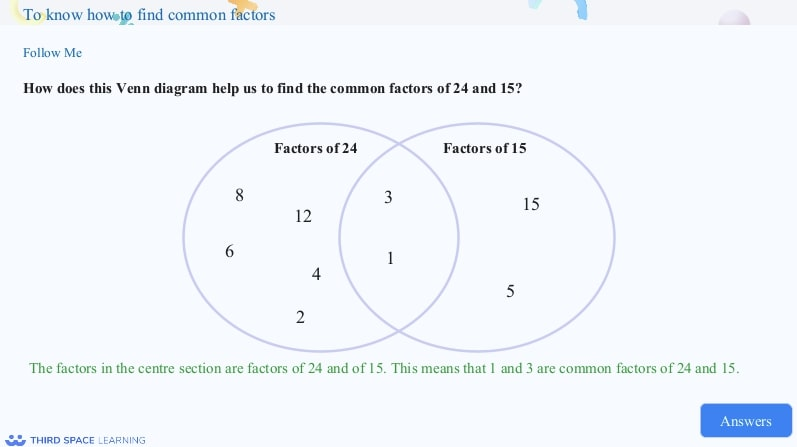Year 5 pupils build upon their knowledge of factors by finding common factors of numbers.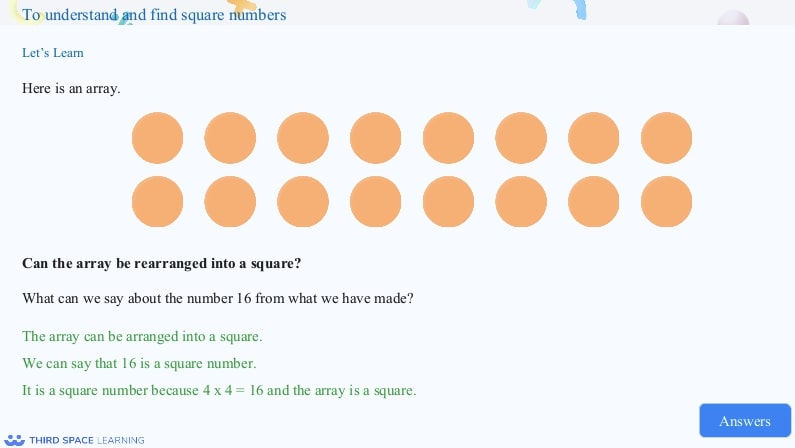Pupils should be familiar with arrays when they are in Year 5. They can use their understanding of arrays to explore square numbers.

#### Example year 5 multiplication and division A questions

1. Find the common factors for 12 and 36

Answer: 1, 2, 3, 4 and 6

2. Multiply 23 by 10, 100 and 100

23 x 10 = 230

23 x 100 = 2300

23 x 1000 = 23,000

### Fractions Year 5

Fractions are also split into two blocks, with Block A covered at the end of the autumn term and Block B in the spring term.

### Fractions A Year 5

Fractions A is the first of the two fraction blocks taught in Year 5. Pupils continue to develop their understanding of equivalent fractions and learn how to convert between improper fractions and mixed numbers. In block A, students build on the skills developed in Year 4; progressing from adding and subtracting fractions to adding adding and subtracting mixed numbers.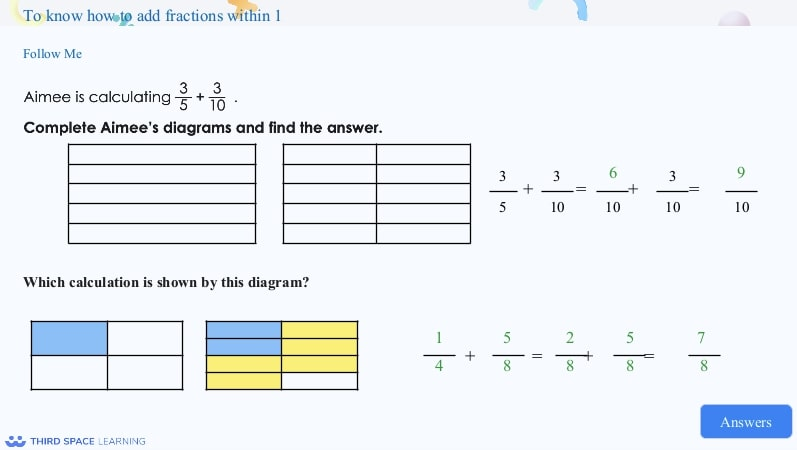Pupils move on from adding and subtracting fractions with the same denominator to adding and subtracting fractions with different denominators. This extends to fractions greater than 1.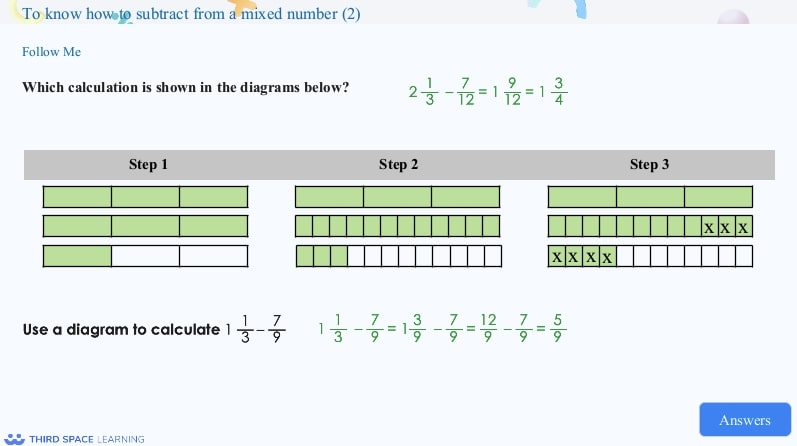This example demonstrates how diagrams can be used to explain how to subtract a fraction from a mixed number.

#### Example year 5 Fractions A questions

1. Give 3 fractions which are equivalent to \frac{2}{3}

Answer: Any fraction where the numerator and denominator have been multiplied by the same amount

E.g. \frac{4}{6}, \frac{6}{9}, \frac{8}{12}, \frac{10}{15}

2.  5\frac{1}{4} + 6\frac{2}{8} =

## White Rose Maths Year 5 spring term

In the spring term, Year 5 focuses on multiplication & division, fractions, decimals & percentages, perimeter & area and statistics.

### Multiplication and Division B Year 5

In the second multiplication and division block,  students spend time developing their skills of formal written methods. For multiplication, they begin by multiplying numbers up to 4-digits by a 1-digit number and progress to multiplying by a 2-digit number. Pupils are introduced to the short division (bus stop) method, initially, focusing on dividing a 4 -digit number by 1-digit, progressing to division with remainders. These formal written methods are then applied to problem solving situations.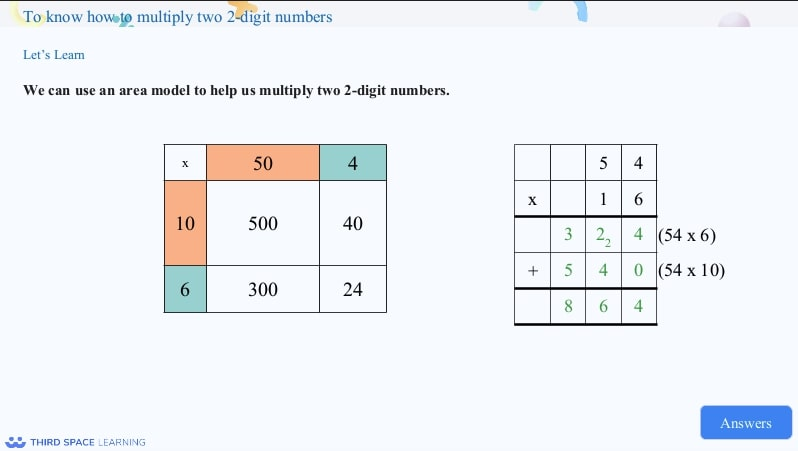This example shows pupils moving from the area model to a formal written method of multiplication.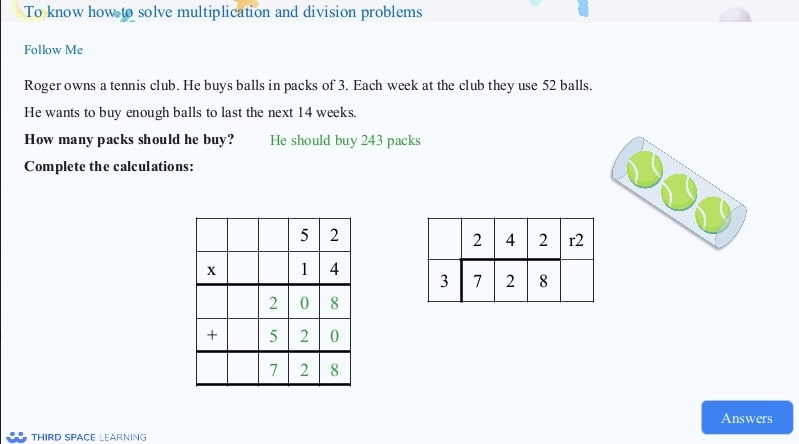Pupils are given a chance to apply their understanding of multiplication and division through problem solving.

1. 6432 x 54 =

2. 7564 ÷ 6

### Fractions B Year 5

In this second, shorter fractions block, pupils learn to multiply fractions; initially multiplying fractions by integers, progressing to multiplying mixed numbers by integers. Students also continue to build on their skills of calculating fractions of amounts.

#### Example year 5 fractions B questions

1. Calculate \frac{3}{5} of 270

### Decimals and percentages Year 5

In Year 5, pupils are exposed to decimals in the spring term, but again in a topic of its own in the summer term. Pupils learn to make connections between fractions, decimals and percentages. Students begin by investigating the equivalence between fractions and decimals as tenths, hundredths and thousandths, followed by ordering and comparing decimals up to 3 decimal places and rounding decimals to the nearest whole number. Pupils are first introduced to percentages in Year 4. This is built upon Year 5, when they really develop their understanding of the link between percentages, decimals and fractions.

#### Example year 5 decimals and percentages questions

1. Write \frac{42}{200} as a decimal and a percentage

2. Order the following numbers from smallest to largest

53.23          53.2          53.03                53.3

### Perimeter and area  Year 5

Perimeter and area is a short topic in Year 5. Pupils build on the knowledge gained in Year 4, to measure and calculate the perimeter of composite rectilinear shapes and to calculate the area of rectangles and other irregular shapes.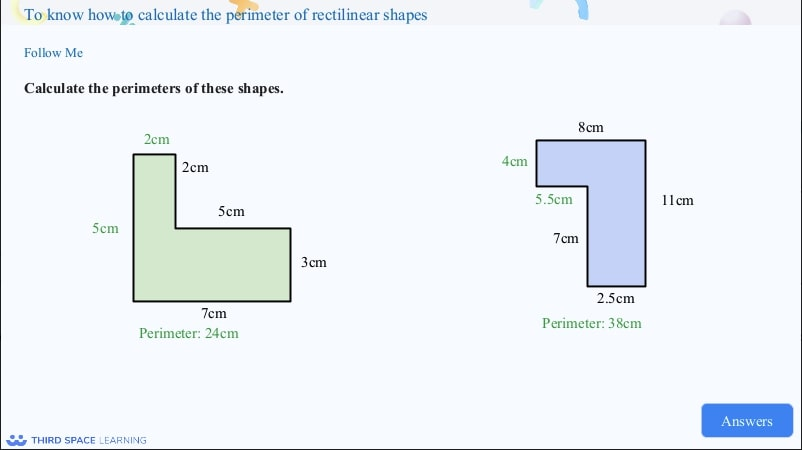Pupils will have looked at finding the perimeter of rectilinear shapes in Year 4. This is continued in Year 5, including where rectilinear shapes have multiple missing sides, including side with decimals.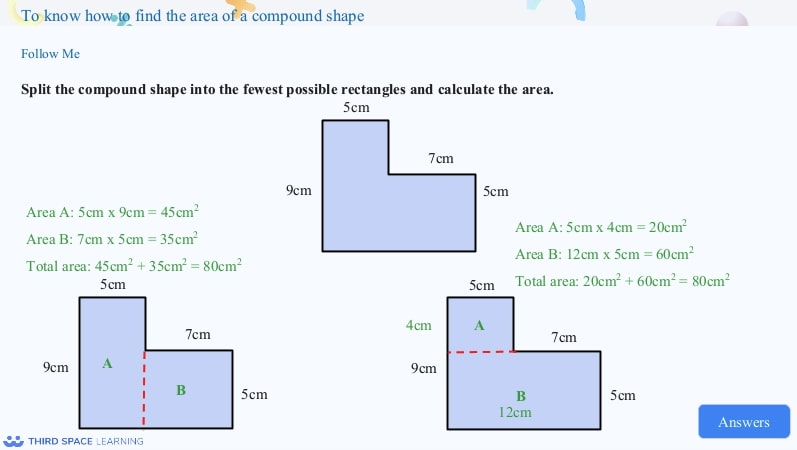Pupils extend their understanding of area by finding the area of compound shapes.

#### Example year 5 perimeter and area questions

1. A regular hexagon has sides which are 7.5cm in length. What is the perimeter of the hexagon?

2. A kitchen is 6.5m long and 3m wide. What is its area?

### Statistics  Year 5

In Year 5, pupils build on the statistics work completed in Year 4; connecting their work on coordinates and scales to their interpretations of time graphs. At this stage pupils are also introduced to reading and interpreting timetables.

#### Example year 5 statistics questions

1 .Monthly rainfall was measured for a year. Results are recorded in the table below. Use these results to create a line graph.

## White Rose Maths Year 5 summer term

In the summer term, Year 5 focuses on shape, position & direction, decimals, negative numbers, converting units and volume

### Shape Year 5

In summer block 1 of Year 5, pupils become more accurate in drawing lines with a ruler to the nearest millimetre and measuring with a protractor. Students learn to identify angles at a point and one whole turn, and angles at a point and on a straight line. Pupils use the properties of rectangles to deduce facts about missing lengths and angles and distinguish between regular and irregular polygons based on reasoning about equal sides and angles. Students also become more familiar with 3D shapes.

#### Example year 5 shape questions

1. Calculate the size of angle a

2. Explain why a square is a regular polygon.

Answer: A square has all sides the same length and all angles the same size.

### Position and Direction  Year 5

In Year 5, pupils recognise and use reflection and translation in a variety of diagrams. They build on their knowledge from Year 4; continuing to use a 2D grid and coordinates in the first quadrant and translating with coordinates. Students also continue to build on their understanding of symmetry; reflecting in horizontal and vertical lines.

#### Example year 5 position and direction questions

1.Plot the following coordinates on a grid:

(2,3) (4,3) and (4,5)

2. What coordinates would you need to plot to make a square?

### Decimals  Year 5

The summer term is the second time pupils are exposed to decimals. In this topic, pupils add and subtract decimals; initially with the same number of decimal places, but then progressing to different numbers of decimal places. Students also multiply whole numbers and decimals by 10, 100 and 1000.

#### Example year 5 decimals questions

1. Multiply 0.34 by 10, 100 and 1000

0.34 x 10 = 3.4

0.34 x 100 = 34

0.34 x 1000 = 340

2. 7.34 – 5.368 =

### Negative Numbers  Year 5

This is a very short block, in which pupils begin to understand negative numbers. They begin by counting through zero in 1s, followed by counting through zeros in multiples. Students also compare and order negative numbers and find the difference.

#### Example year 5 negative number questions

1. 3 – 14 =

2. What is the difference between -17 and 8

### Converting Units  Year 5

In Year 5, pupils convert between a range of units; including kilograms and kilometres; millimetres and centimetres; centimetres and metres; grams and kilograms and litres and millilitres. In this block, students also convert units of time and calculate with timetables.

#### Example year 5 converting units questions

1. 5.34kg = ______g

2. A film starts at 7:25pm and lasts for 1 hour and 35 minutes. What time does it finish? Give your answer in 24 hour clock.

### Volume  Year 5

This is a very short final block in Year 5. Pupils learn about cubic centimetres and comparing volume. They also estimate volume and capacity.

#### Example year 5 volume questions

1. What is the volume of the shape below, if each cube is 1cm x 1cm x 1cm?

Do you have pupils who need extra support in maths?
Every week Third Space Learning’s maths specialist tutors support thousands of pupils across hundreds of schools with weekly online 1-to-1 lessons and maths interventions designed to plug gaps and boost progress.

Since 2013 we’ve helped over 150,000 primary and secondary school pupils become more confident, able mathematicians. Learn more or request a personalised quote for your school to speak to us about your school’s needs and how we can help.

Subsidised one to one maths tutoring from the UK’s most affordable DfE-approved one to one tutoring provider.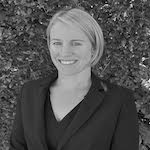##### Emma Johnson
Emma is a former Deputy Head Teacher, with 12 years' experience leading primary maths. Over the past 18 years, she has worked in primary schools in the UK and internationally, in Qatar. She now runs a tutoring company and writes resources and blogs for Third Space Learning, She is also the creator of the YouTube channel Maths4Kids with her daughter, Amber.

Related Articles

x#### Number and Place Value Diagnostic Quiz Year 5

Download this free number and place value diagnostic quiz for year 5 to identify gaps and growth in learning.

#### Number and Place Value Diagnostic Quiz Year 5Download this free number and place value diagnostic quiz for year 5 to identify gaps and growth in learning.